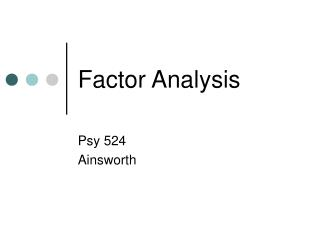DownloadDownload PresentationFactor Analysis

Factor Analysis

Télécharger la présentationFactor Analysis

- - - - - - - - - - - - - - - - - - - - - - - - - - - E N D - - - - - - - - - - - - - - - - - - - - - - - - - - -
Presentation Transcript

1. Factor Analysis Psy 524 Ainsworth

2. What is Factor Analysis (FA)? • FA and PCA (principal components analysis) are methods of data reduction • Take many variables and explain them with a few “factors” or “components” • Correlated variables are grouped together and separated from other variables with low or no correlation

3. What is FA? • Patterns of correlations are identified and either used as descriptives (PCA) or as indicative of underlying theory (FA) • Process of providing an operational definition for latent construct (through regression equation)

4. What is FA? • FA and PCA are not much different than canonical correlation in terms of generating canonical variates from linear combinations of variables • Although there are now no “sides” of the equation • And your not necessarily correlating the “factors”, “components”, “variates”, etc.

5. General Steps to FA • Step 1: Selecting and Measuring a set of variables in a given domain • Step 2: Data screening in order to prepare the correlation matrix • Step 3: Factor Extraction • Step 4: Factor Rotation to increase interpretability • Step 5: Interpretation • Further Steps: Validation and Reliability of the measures

6. “Good Factor” • A good factor: • Makes sense • will be easy to interpret • simple structure • Lacks complex loadings

7. Problems w/ FA • Unlike many of the analyses so far there is no statistical criterion to compare the linear combination to • In MANOVA we create linear combinations that maximally differentiate groups • In Canonical correlation one linear combination is used to correlate with another

8. Problems w/ FA • It is more art than science • There are a number of extraction methods (PCA, FA, etc.) • There are a number of rotation methods (Orthogonal, Oblique) • Number of factors to extract • Communality estimates • ETC… • This is what makes it great…

9. Problems w/ FA • Life (researcher) saver • Often when nothing else can be salvaged from research a FA or PCA will be conducted

10. Types of FA • Exploratory FA • Summarizing data by grouping correlated variables • Investigating sets of measured variables related to theoretical constructs • Usually done near the onset of research • The type of FA and PCA we are talking about in this chapter

11. Types of FA • Confirmatory FA • More advanced technique • When factor structure is known or at least theorized • Testing generalization of factor structure to new data, etc. • This is tested through SEM methods discussed in the next chapter

12. Terminology • Observed Correlation Matrix • Reproduced Correlation Matrix • Residual Correlation Matrix

13. Terminology • Orthogonal Rotation • Loading Matrix – correlation between each variable and the factor • Oblique Rotation • Factor Correlation Matrix – correlation between the factors • Structure Matrix – correlation between factors and variables • Pattern Matrix – unique relationship between each factor and variable uncontaminated by overlap between the factors

14. Terminology • Factor Coefficient matrix – coefficients used to calculate factor scores (like regression coefficients)

15. FA vs. PCA conceptually • FA produces factors; PCA produces components • Factors cause variables; components are aggregates of the variables

16. Conceptual FA and PCA

17. FA vs. PCA conceptually • FA analyzes only the variance shared among the variables (common variance without error or unique variance); PCA analyzes all of the variance • FA: “What are the underlying processes that could produce these correlations?”; PCA: Just summarize empirical associations, very data driven

18. Questions • Three general goals: data reduction, describe relationships and test theories about relationships (next chapter) • How many interpretable factors exist in the data? or How many factors are needed to summarize the pattern of correlations?

19. Questions • What does each factor mean? Interpretation? • What is the percentage of variance in the data accounted for by the factors?

20. Questions • Which factors account for the most variance? • How well does the factor structure fit a given theory? • What would each subject’s score be if they could be measured directly on the factors?

21. Considerations (from Comrey and Lee, 1992) • Hypotheses about factors believed to underlie a domain • Should have 6 or more for stable solution • Include marker variables • Pure variables – correlated with only one factor • They define the factor clearly • Complex variables load on more than on factor and muddy the water

22. Considerations (from Comrey and Lee, 1992) • Make sure the sample chosen is spread out on possible scores on the variables and the factors being measured • Factors are known to change across samples and time points, so samples should be tested before being pooled together